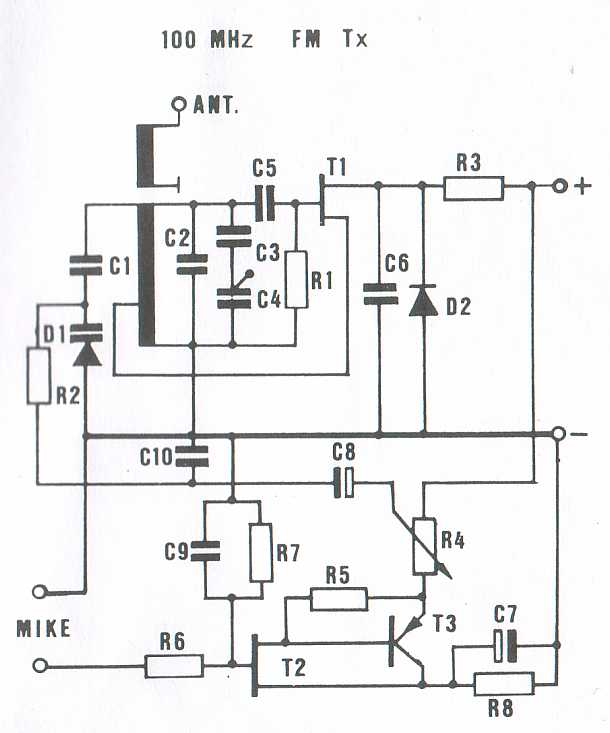# FM Modulator## Partlist:

D1 = Varicap
D2 = 1N4148
R1 = 100kOhm
R2 = 220kOhm
R3 = 22Ohm
RV4 = 1kOhm
R5 = 1kOhm
R6 = 56kOhm
R7 = 1mOhm
R8 = 1,2kOhm
C1 = 5pF
C2 = 6pF
C3, C5 = 15pF
C4 = trimmer
C6 = 1nF Ceramic
C10 = 1nF Ceramic
C8 = 4,7uF
C9 = 100pF Ceramic
T1 = BF245A or BF245B FET
T2 = 2N3819 FET
T3 = BC307-8-9 or BC557-8-9
C7 = 100uF